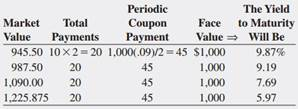### Create an Account

Home / Questions / Using a Spreadsheet to Calculate Yield to Maturity What is the yield to maturity on the fo...

# Using a Spreadsheet to Calculate Yield to Maturity What is the yield to maturity on the following bonds all have a maturity of 10 years a face value of \$1000 and a coupon rate of 9 percent

Using a Spreadsheet to Calculate Yield to Maturity. What is the yield to maturity on the following bonds; all have a maturity of 10 years, a face value of \$1,000, and a coupon rate of 9 percent (paid semiannually). The bonds’ current market values are \$945.50, \$987.50, \$1,090.00, and \$1,225.875, respectively. ( LG 3-2 )( LG 3-2 )

Bond Valuation Formula Used to Calculate Fair Present Values

Most bonds pay a stated coupon rate of interest to the holders of the bonds. These bonds are called coupon bonds. The interest, or coupon, payments per year, INT, are generally constant (are fixed) over the life of the bond. 5 Thus, the fixed interest payment is essentially an annuity paid to the bond holder periodically (normally semiannually) over the life of the bond. Bonds that do not pay coupon interest are called zero-coupon bonds. For these bonds, INT is zero. The face or par value of the bond, on the other hand, is a lump sum payment received by the bond holder when the bond matures. Face value is generally set at \$1,000 in the U.S. bond market.

May 18 2020 View more View LessSubscribe To Get Solution